Latest Banking jobs   »

# Quantitative Aptitude Quiz For RBI Grade B/ ECGC PO/ SIDBI Grade A Prelims 2022- 15th May

Directions (1-5): In a coaching class total no. of students are 336 and each student likes at least one subject out of three subjects i.e. (quant, reasoning and English). No. of students who like only quant are 60 and no. of students who like reasoning with at most one more subject are 133⅓% more than no. of students who likes only English. Total no. of students who like all three subjects are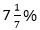of total students of the class and ratio of students who like both quant and English only, no. of students who like both reasoning and English only and student who like both quant and reasoning only are 1:3:2. Total no. of students who like at least two subjects are 96.

Q1. Find no. of students who likes only reasoning?
(a) 108
(b) 124
(c) 112
(d) 144
(e) 132

Q2. What is the ratio of students who like both quant and reasoning only to students who like all the subjects together?
(a) 1:2
(b) 2:3
(c) 1:1
(d) 2:1
(e) None of these.

Q3. No. of students who likes both quant and English are what percent of students who likes only English?
(a) 25%
(b) 20%
(c) 40%
(d) 33.33%
(e) 50%

Q4. What is the average no. of students who likes only quant, only English and only reasoning?
(a) 72
(b) 60
(c) 80
(d) 100
(e) 75

Q5. No. of students who like both reasoning and English are what percent of students who like English?
(a) 41.33%
(b) 20%
(c) 33.33%
(d) 33.67%
(e) 41 2/3%

Directions (6-10): What will come in place of question mark (?) in the following number series?

Q6. 7.5, 41, 81, 129.5, 190.5, ?
(a) 275
(b) 270
(c) 271.5
(d) 272.5
(e) 269.5

Q7. 13, 113, 775, 3839, ?, 11353
(a) 11417
(b) 7853
(c) 11253
(d) 11453
(e) 19131

Q8. 122, 166.5, 205.5, 255.5, 283.5, ?
(a) 523
(b) 483.5
(c) 355.5
(d) 393.5
(e) 423.5

Q9. 620 632 608 644 596 ?
(a) 536
(b) 556
(c) 656
(d) 646
(e) None of these

Q10. 572, ?, 1012, 1280, 1580, 1912
(a) 476
(b) 668
(c) 776
(d) 772
(e) 1016

Directions (11-15): In the following questions, two quantities (I) and (II) are given. You have to solve both the quantities and mark the appropriate answer.

Q11. Quantity I: Anurag invested Rs.3000 more than that of Veer, while ratio of investment period for Anurag and Veer is 5 : 6 respectively. If out of total profit of Rs.27360, Veer got Rs.12960, then find total investment done by Anurag.
Quantity II: Shivam and Deepak started a business by investing Rs.25000 and Rs.10000 respectively. At the end of the year, they decided to divide 60% of the total profit equally and rest in the investment ratio. If they had divided total profit in investment ratio, then Shivam will receive Rs.9000 more profit than that of he received actually, then find the difference between actual profit share of Shivam and that of Deepak?
(a) Quantity I < Quantity II
(b) Quantity I ≤ Quantity II
(c) Quantity I > Quantity II
(d) Quantity I ≥ Quantity II
(e) Quantity I = Quantity II or no relation.

Q12. Quantity I: Aman invested Rs.100000 at 16% p.a. The interest was compounded half yearly in the first year and in the second & third year it was compounded yearly. What will be the total interest received by man at the end of 3 years?
Quantity II: The simple interest received on a sum of Rs.2,30,000 at the rate of 23% p.a. in N years is Rs.4,23,200. Find the CI received on the same sum in (N/4) years at the rate of 11% p.a. compounded annually.
(a) Quantity I < Quantity II
(b) Quantity I ≤ Quantity II
(c) Quantity I > Quantity II
(d) Quantity I ≥ Quantity II
(e) Quantity I = Quantity II or no relation.

Q13. Quantity I: The ratio of speed of boat in still water to the speed of stream is 9 : 5. A boat takes total 7 (7/8) hours to travel 98 km each in upstream and in downstream. Find the speed of boat is still water.
Quantity II: Ratio of length of train – A to that of train – B is 3 : 4. Train – A crosses train – B in 48 seconds while running in same direction and train – B crosses a pole in 16 seconds. If train – A crosses a 520m long platform in 24 seconds, then find difference in speed of train – A & B. (in km/hr.)
(a) Quantity I < Quantity II
(b) Quantity I ≤ Quantity II
(c) Quantity I > Quantity II
(d) Quantity I ≥ Quantity II
(e) Quantity I = Quantity II or no relation.

Q14. Quantity I: Ratio of cost price of two articles – A & B is 5 : 6 respectively and shopkeeper marked article – A & B 40% and 25% above cost price respectively. If shopkeeper allows discount of 25% on A and 10% on B, then he get a total profit of Rs.300. Find the total cost price of article A & B.
Quantity II: Selling price of an article sold by Amit and Arun is same. Amit sold article at 20% profit and Arun sold at 25% loss. Amit calculated profit percent at cost price whereas Arun calculated loss percent at selling price. If total loss of Amit and Arun together is Rs.200. Find total cost price of Amit’s article and Arun’s article ?
(a) Quantity I < Quantity II
(b) Quantity I ≤ Quantity II
(c) Quantity I > Quantity II
(d) Quantity I ≥ Quantity II
(e) Quantity I = Quantity II or no relation.

Q15. Quantity I: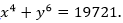If y=5 and x & y are positive integers, then find x is what percent of y.
Quantity II: A is 20% less than B and B is 10% less than C. If ratio of A to D is 4: 5, then find A & D together are what percent of C.
(a) Quantity I < Quantity II
(b) Quantity I ≤ Quantity II
(c) Quantity I > Quantity II
(d) Quantity I ≥ Quantity II
(e) Quantity I = Quantity II or no relation.

Solutions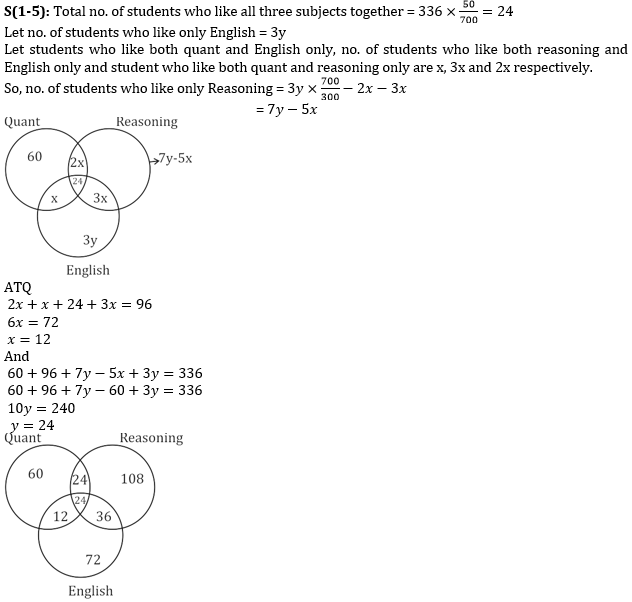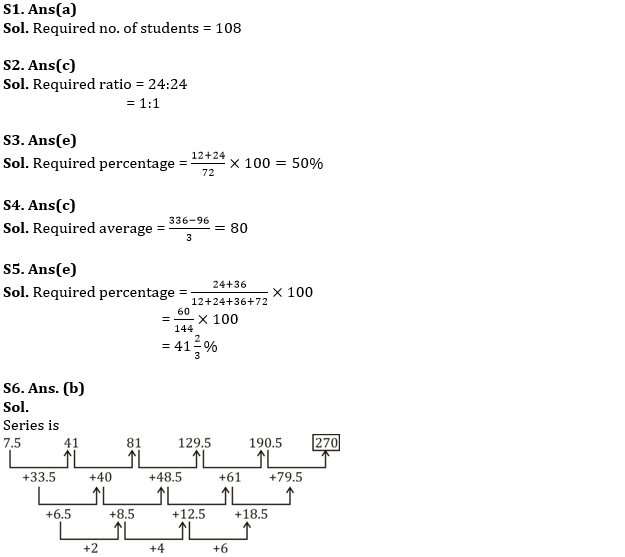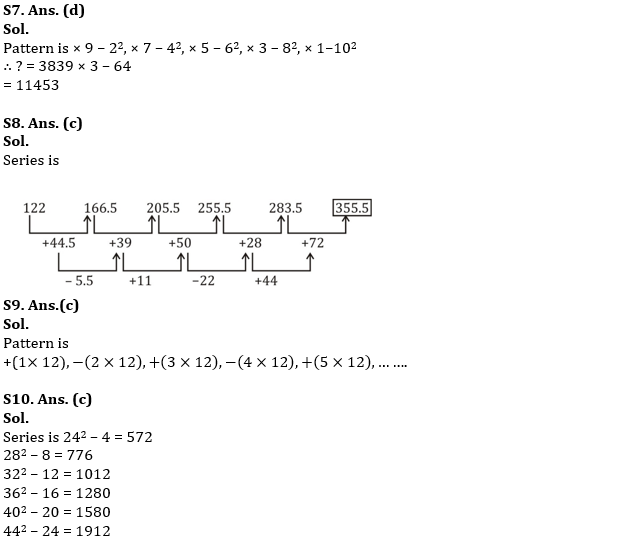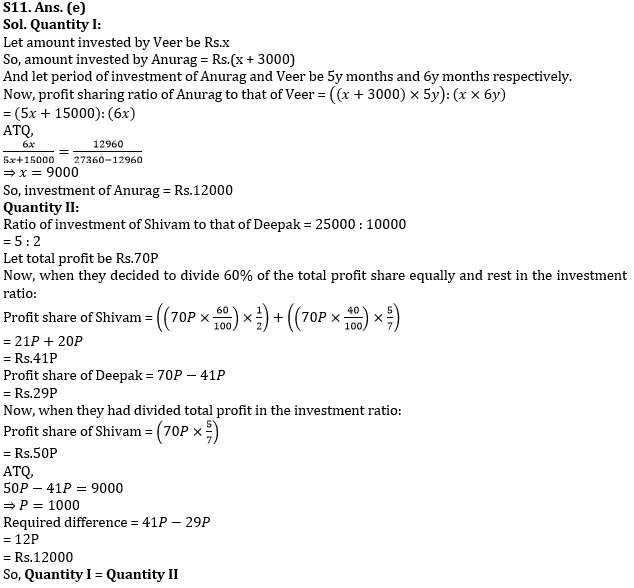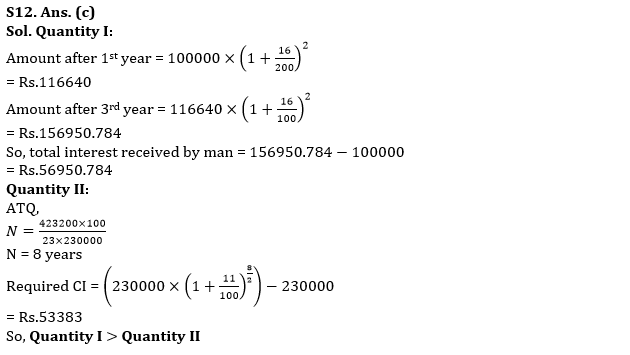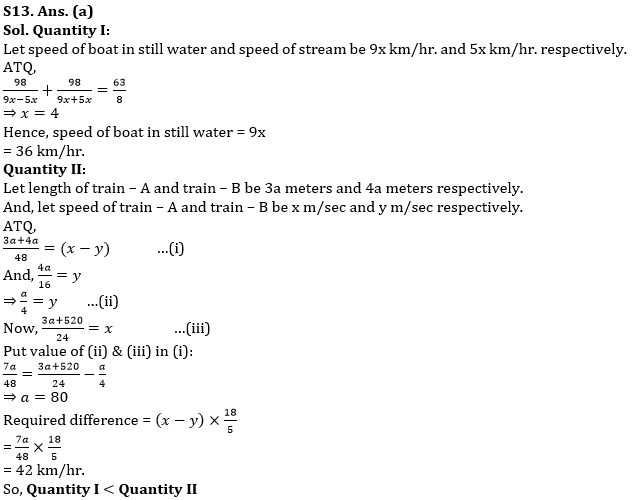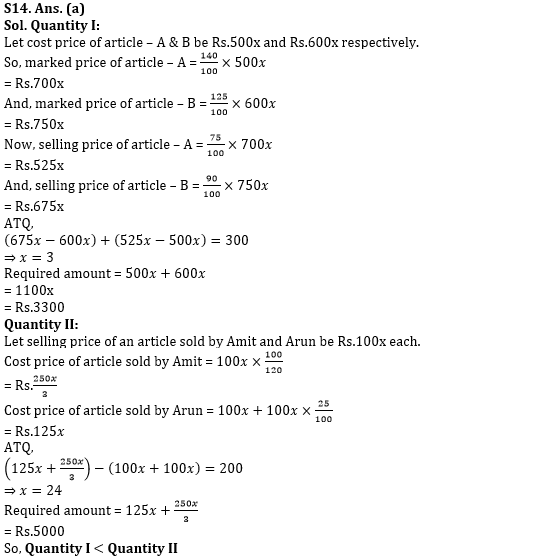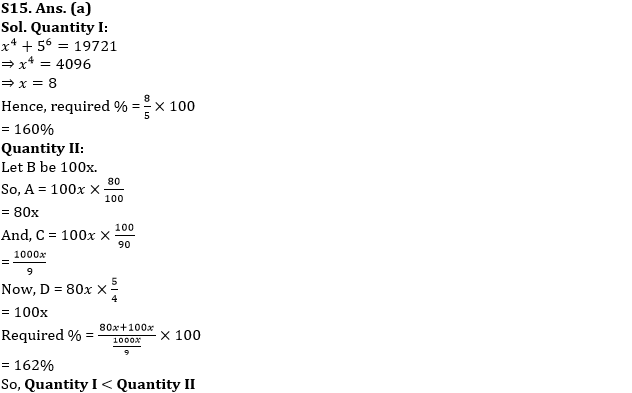#### Congratulations!